# Effect of ZnO Nanoparticles on the Thermo-Physical Properties and Heat Transfer of Nano-Fluid Flows

Effect of ZnO Nanoparticles on the Thermo-Physical Properties and Heat Transfer of Nano-Fluid Flows
Chemical Engineering Department, Faculty of Engineering, University of Kufa, Najaf 54002, Iraq

Corresponding Author Email:
asmaah.alhusseini@uokufa.edu.iq
Page:
715-721
|
DOI:
https://doi.org/10.18280/ijht.380316
22 February 2020
|
Accepted:
30 August 2020
|
Published:
15 October 2020
| Citation

OPEN ACCESS

Abstract:

In this research, an improvement in alternative heat transfer and thermal properties of ZnO nanoparticles (NPs)/ distilled water, DW nanofluid are experimentally studied and investigated. The two-step approach is utilized to prepare the ZnO NPs/water nanofluid using different concentrations and different inlet temperatures. Scanning Electron Microscopy (SEM) and UV–visible spectrophotometer is employed to characterize the nanofluid. Varying flow rates and the temperatures are examined in the heat exchanger's horizontial tube and shell pipe as well as the impacts of ZnO nanoparticles and the temperatures are studied on nanofluid's thermal conductivity and viscosity. The findings show improvements in the thermal conductivity of ZnO NPs / DW nanofluid. A maximum value of 6.67% of the thermal conductivity is achieved at a temperature of 343K using 1 % ZnO NPs. Nusselt Number also shown an improvement of 38% at a temperature of 343K using 0.2% wt of ZnO NPs under the turbulent condition (Reynolds Number range of 8000 to 20000). Experimental results are compared with previous correlations and an acceptable agreement is observed.

Keywords:

nanofluid, heat transfer, Nusselt number, ZnO nanoparticles, flow rate, thermal conductivity, viscosity

1. Introduction

To boost the heat transfer in heat exchangers, it is necessary of finding ways to enhance the properties of the fluids. Nanoparticles are used to improve heat transfer by influence the characteristics of traditional fluid such as water and the resulting mixture is called nanofluid. These types of fluids are usually having the combined merits of both base fluid and nanoparticles [1-3]. Therefore, it is very vital to prepare the nanofluids and study its thermal and physical properties due to the limitation of measurement techniques of heat transfer .

Several studies were employed with various types of nanoparticles as a passive technique to enhance heat transfer. Kumar et al.  investigated the properties of heat transfer and pressure drop of the CuO- Distilled water nanofluid in a horizontal circular pipe were conducted. Their results showed an increase in the characteristics of base fluid after addition to CuO nanoparticles such as thermal conductivity, viscosity, density, and specific heat. The heat transfer coefficient increased to 46.1 % at 0.5 vol. %, 20 LPH (liter per hour) flow rate, and the Friction factor were drops with the rising inflow rate. Xie et al.  worked on a range of nanofluid forms including TiO2, ZnO, Al2O3, and MgO in a fluid of 55%water and 45%ethylene glycol. At a steady wall temperature, the laminar stream was flowing into a circular copper pipe. An increase in convective heat transfer at a Reynolds number of 1000 to 252% of MgO has been achieved. Ajeel et al. [7-9] utilized SiO2 nanoparticles to analyze the thermal performance of corrugated channels. The findings highlighted new correlations for used SiO2-water nanofluid in terms of Nusselt number and friction factor.

Many researchers utilized ZnONPs in their work with different boundary conditions and applications. Nemade  noticed that ZnO nanoparticles with water nanofluid representing a rise in heat transfer at different nanoparticles concentration. in addition, the concluded study that the ratio of thermal conductivity is more significant than unity which made it preferred in heat exchangers applications.

An increase to about 136% at 50°C in thermal conductivity was observed and in Nusselt number development for ZnO volume concentration of 0.25% in the nanofluid . Yu et al.  prepared nanofluid EG (Ethylene Glycol) / ZnO and recorded an increase in nanofluid thermal conductivity of 26.5% at 5% concentration. Mourgues et al.  stated that due to the effect of ZnO nanofluid, the critical heat flux increased by 54%. Jeong et al.  analyzed the impact of the shape of ZnO nanoparticles in terms of thermal conductivity and their findings showed that the thermal conductivity increase was 18% and 12% for rectangular and spherical shaped ZnO nanoparticles, respectively.

Suganthi et al.  prepared and compared two types of nanofluids depend on using ZnO nanoparticles in terms of thermal conductivity. The outcomes uncovered that increase in thermal conductivity was 33.4% and 17.26% when used ZnO particles with Ethylene Glycol (EG) and water-EG as base fluid, respectively. Suganthi and Rajan  employed ZnO-propylene glycol nanofluids to examine the heat transfer characteristics. the study said that the heat transfer improvement was 4.24% due to effect 2 vol% of tested nanoparticles. Esfe et al. [17-19] studied rheological behavior of hybrid nanofluid and suggested a new experimental correlation to predict the viscosity of the nanofluid at different temperatures and the solid volume fraction.

Recently, Thermo-physical properties measurements, such as thermal conductivity and viscosity, of ZnO in two type propylene glycol (PG) and ethylene glycol (EG) mixed with water, showed an enhancement of 15% and 9% correspondingly. Those researches have done by Akbarzadeh et al.  and they proposed new models for predicting the influential viscosity and thermal conductivity.

ZnO nanoparticle was used to overcome several common cases such as its lower cost and high dispersion ability compared to other materials and It can be called zinc oxide multi-functional material with its physical and chemical properties unique.

Based on the above literature, the emphasis was given to only the impact of ZnO nanoparticles in the thermal transport phenomenon, while the major parameters affecting ZnO behavior such as thermophysical properties, nanofluid preparation, and inlet temperature have left intact. Accordingly, the current study would take into account the effect of viscosity and thermal conductivity at various concentrations, besides the entrance temperatures and flow rates to clarify their impact on the overall thermal performance of ZnO nanoparticles.

2. Nanofluid Preparation

ZnO/ DW nanofluid containing ZnO powder of particle size 53nm and distilled water (DW) is prepared by two-step method at different concentrations of 0.2, 0.4, 0.6, 0.8 and 1 wt%, the reason for selecting these certain volume concentrations (0.2,0.4,0.6,0.8 and 1%) of ZnO/water because of the minimum occurrence of aggregation, stable and homogeneous with this volume concentration compared with high concentrations. Note that there is no sedimentation after 7 days when used lower concentrations of nanofluid but with higher concentrations than above 1%, the solution has begun sedimentation and noticed its thermophysical properties drop.

An ultrasonic homogenizer of 1200 W is run for 30mins to ensure maximum dispersion ZnO nanoparticles in DW. ZnO NPs are ordered from USA nanomaterials co. (www.us-nano.com) and DW is obtained from a local supplier. Table 1 shows the ZnO NPs and DW physical properties utilized in this research.

The UV–V absorption optical properties of the prepared samples are measured using UVWIN 5 Spectrophotometer. Thermal conductivity is measured using the KD2 Pro from Decagon devices. The Viscosity of the samples is measured using a Fungilab viscometer instrument. Figure 1 shows the measuring thermal conductivity of the ZnO NPs/DW sample by KD2 Pro.

## 1.pngFigure 1. Thermal conductivity measuring of ZnO/DW nanofluids by KD2 Pro

## 2.pngFigure 2. UV-Visible absorption spectrum of ZnO/DW nanofluids

## 3.png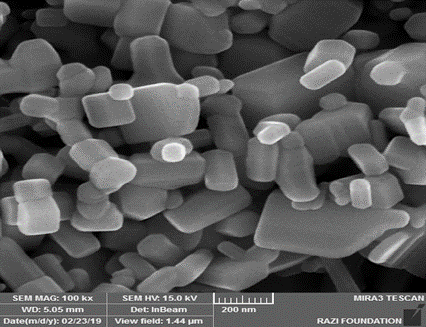Figure 3. SEM of ZnO NPs

The UV–visible absorption spectrum of ZnO NPs/DW is measured in the wavelength range of 350 - 400 nm. Figure 2 shows the absorbance spectrum of the ZnO nanoparticles. The band edges of absorption are recorded at a peak wavelength of 376 nm.

Figure 3 shows the SEM images ZnO nanoparticles. It is observed that the ZnO NPs have irregular morphology with an average size ranged between 30-50nm. Also, particle morphology is hexagonal.

3. Experimental Description

The experimental devices used in the current study are shown in Figure 4. It involves nanofluid and water flow loops, heating unit to heat the nanofluid solution, temperature measurement system, chiller, a couple of pumps of types centrifugal pump so as to outfit needful flow rates, two flow meters, reads the flow rate amount that required to be based on the wanted Reynold number, and valves to control the flow rates.

Table 1. Material characteristics

 Material Average particle diameter, nm Purity, % Density kg/m3 Heat capacity J/kg.k ZnO 53 99.9% 5606 523 Water - - 995 4179

## 4.pngFigure 4. Experimental rig for current study

The heat exchanger is constructed from copper with 14×16 (22.4) mm diameter of tube and 48.5 mm diameter of shell and 355 mm length, making external insulation for the pipe to reduce system heat losses by using a layer of metal wool and aluminum foil. The test unit is heated electrically by a 6 kW DC power. A six-liter capacity chiller is utilized to preserve the fixed temperature in the inlet section of the test. The temperature measurements were taken at the entrance and exit position of fluid until steady-state has arrived. When the temperatures were stable with time approximately 15 min, a steady-state was established. Many experimental carried out at different temperatures of 303, 323, and 343 K and flow rates of 100, 200, and 300 L/h. Heat exchange takes place in the test chamber and the differences of temperature between inlet and outlet of the working fluid flows were calculated. All experiments are carried out using a parallel-flow tube-shell horizontal heat exchanger because of the advantage of parallel is that a small diameter pipe can be applied due to decrease pressure declines and when two fluids are wanted to be brought to about the same temperature.

The following relationships are used to estimate thermophysical properties and heat transfer of nanofluid:

$c p_{n f}=\varphi c p,_{p}+(1-\varphi) c p,_{f}$           (1)

$Q_{n f}=m^{.} c p\left(T_{\circ}-T_{i}\right)$            (2)

$\Delta T_{L M T D}=\frac{\left(T_{i, \text {shell}}-T_{\text {O,tube}}\right)-\left(T_{\text {O,shell}}-T_{\text {O,tube}}\right)}{L N\left(T_{\text {i,shell}}-T_{\text {O,tube}}\right) /\left(T_{\text {O,shell}}-T_{\text {O,tube}}\right)}$           (3)

$h=\frac{Q_{n f}}{\Pi d L \Delta T_{L M T D}}$           (4)

$R e=\frac{\rho u d_{i}}{\mu}$           (5)

$N u=\frac{h d}{k}$          (6)

4. Uncertainty

When conducting tests in the laboratory, there will be errors regardless of the thoroughness of followed procedures or algorithms because each device or instrument has its own uncertainty, besides there is no device without a specific error. Uncertainties were calculated for the variables in this study at a 95% confidence level as Coleman and Steele reported  as follows:

$\left(\frac{\delta Q}{Q}\right)=\sqrt{\left[\left(\frac{\delta U}{U}\right)^{2}+\left(\frac{\delta D}{D}\right)^{2}+\left(\frac{\delta \rho}{\rho}\right)^{2}+\left(\frac{\delta T}{T}\right)^{2}+\left(\frac{\delta C P}{C P}\right)^{2}+\left(\frac{\delta \emptyset}{\emptyset}\right)^{2}\right]}=2.2 \%$             (7)

$\left(\frac{\delta R e}{R e}\right)=\sqrt{\left[\left(\frac{\delta U}{U}\right)^{2}+\left(\frac{\delta D}{D}\right)^{2}+\left(\frac{\delta \rho}{\rho}\right)^{2}+\left(\frac{\delta \mu}{\mu}\right)^{2}\right]}= 3.7 \%$                (8)

$\left(\frac{\delta N u}{N u}\right)=\sqrt{\left[\left(\frac{\delta h}{h}\right)^{2}+\left(\frac{\delta D}{D}\right)^{2}+\left(\frac{\delta k}{k}\right)^{2}\right]}=2.0 \%$        (9)

In the above equations, $\emptyset$ calculated from $\emptyset=V_{p} / V_{t}$. Where $V_{t}$ and $V_{p}$ denote the nanoparticles and total volume respectively.

5. Results and Discussion

Figure 5 and Table 2 show the relation between thermal conductivity and temperature of different concentrations of ZnO NPs/water nanofluid.

## 5.png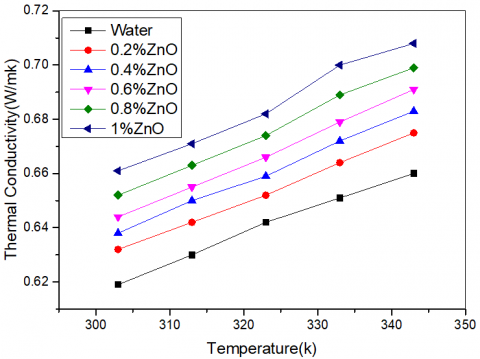Figure 5. The thermal conductivity of ZnONPs/DW nanofluids against temperatures at different ZnONPs nanoparticle volume concentration

Accordingly, the thermal conductivity increases as the concentration of ZnO NPs increasing for all tested temperatures. This increase may attribute to the presence of the suspended particles. In general, several conditions, such as nanofluid crystal structure, temperature, and concentration, influence the thermal conductivity. For all tested concentrations, all the nanofluids ' thermal conductivity was uncovered to be higher than water (base fluid) as well as the same trend is found for all tested fluids. The increase in thermal conductivity with temperature is credited to Brownian motion for nanoparticles in the fluid. At high temperatures, the particle collision occurred at a higher rate, taking with it more kinetic energy. During the collision, the heat transfer of solid-solid could raise the nanofluid's overall thermal conductivity. Especially, Brownian motions itself a process of diffusive.

Consequently, thermal conductivity improved. An enhancement in the thermal conductivity to 66% at a temperature of 343K with ZnONPs 1% is observed. These findings are in agreement with the research results obtained by Suresh Sagadevan .

Figure 6 demonstrates a comparative study of the obtained measurement results of the thermal conductivities of experiments to the equation of the Maxwell-Garnett equation, as shown in the below equation .

$\frac{k}{k_{n f}}=1+\frac{3(\alpha-1) \emptyset}{\alpha+2-(\alpha-1) \emptyset}$            (10)

$\alpha=\frac{k}{\rho c p}$                  (11)

where, k is thermal conductivity (W/m K), nf refers to Nanofluid and $\emptyset$ refer volume concentration. It seems the experimental results have the same behavior as standard correlations. The average error of 15% was also obtained from the comparison of the results. It was attributed to the non-spherical shape of the ZnO nanoparticle .

## 6.png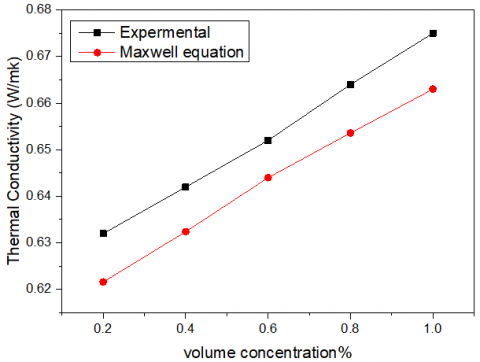Figure 6. Comparison between Maxwell equation and experimental behaviour of thermal conductivity of ZnO NPs

## 7.pngFigure 7. The viscosity of ZnONPs/DW nanofluids against temperatures at different ZnONPs nanoparticle volume concentration

Figure 7 and Table 3 show the viscosity reduction of ZnONPs/DW nanofluid as the temperature rises. This reduction due to the weakening in the intermolecular attraction between nanoparticles and the weakening of the hydrogen-bonding network of the base fluid. The viscosity of nanofluids follows the trend of base fluids, where it decreases exponentially with temperature. However, the increase in viscosity value with increasing ZnO NPs wt% causes an increase in the friction and the resistance of flowing fluids. The viscosity values of the ZnONPs/DW nanofluid obtained at temperature values of 303 and 343K were 1.21 and 0.6 cp respectively at ZnO concentration of 1%. These results are in agreement with the research finding in the paper .

Figure 8 displays the Nusselt number values measured at different flow rates values at three diverse inlet temperature. It is noticed that the amount of heat transferred is positively influenced by the rate of flow. Also, the Nussalt number enhance to about 38% at 343K with 0.2% of ZnO NPs in contrast to the base fluid. These results are in agreement with research findings in the paper . This improvement is attributed to the upgrading in thermal conductivity as a result of the presence of the ZnONPs in the water. As well as, the thickness of the thermal boundary layer could be declined as the mobility of the particles near the wall was neglected at the center of the tube. Besides, viscosity was declined in the region of the wall .

## 8.png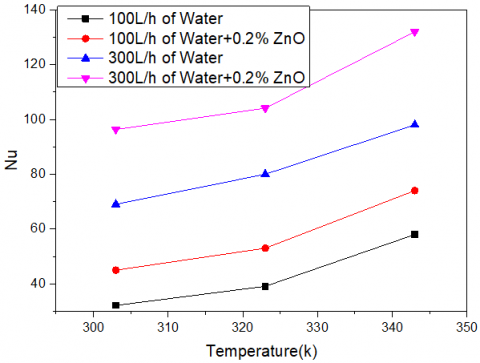Figure 8. The influence of temperature and flow rate of ZnONPs/DW nanofluid on Nusselt number

## 9.png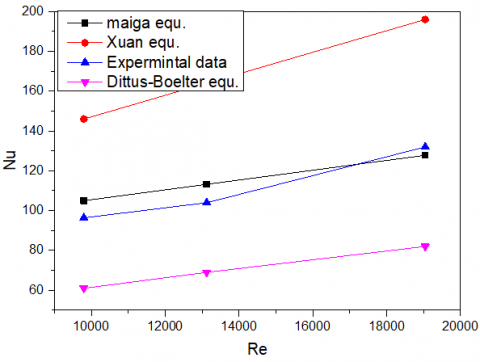Figure 9. The difference between the experiments data and the correlation of the experimental values

Figure 9 illustrates the comparisons between the experimental data and common trial correlations of Xuan et al., Maiga et al. and Dittus-Boelter et al. [27-29], as shown in Eq. (10), Eq. (11) and Eq. (12) respectively. The value of the Nusselt number is increased with increasing Re. The difference between the experimental correlation and experimental data are attributed to nanoparticle clustering. Eq. (8) shows closed data fitting to the experimental data of the current research.

$N u_{n f}=0.0059\left(1+7.6286 \phi^{0.6886} P e_{d}^{0.001}\right) R e_{n f}^{0.9238} \operatorname{Pr}_{n f}^{0.4}$         (12)

$N u_{n f}=0.85 R e_{n f}^{0.71} \operatorname{Pr}_{n f}^{0.35}$           (13)

$N u_{n f}=0.023 R e_{n f}^{0.8} \operatorname{Pr}_{n f}^{0.4}$               (14)

Figure 10 presents the rate of heat transfer (Q) with various flow rates values and various temperatures. As can been seen, as the flow rate increased, the heat transfer rate is increased. For an instant, the temperature increased from 303K to 323K and as a result, the heat transfer rate increased from 287.7 to 447 at 300 L/h. In this way, the same result recorded for increased temperature from 323K to 343K. The best heat transfer rate of 585 (w) is recorded at a high temperature and high flowrate, 343K, and 300 L/h, respectively.

## 10.pngFigure 10. The effect of temperature and flow rate of DW on the Heat transfer rate

## 11.png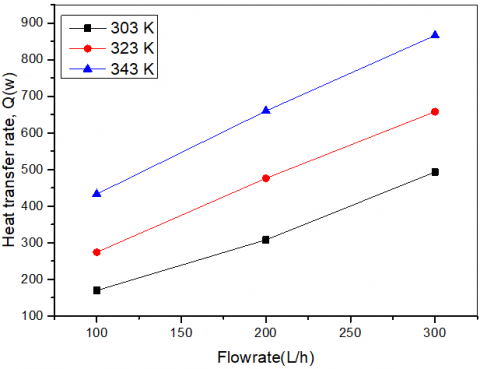Figure 11. The effect of temperature and flowrate of ZnO/water nanofluid on the Heat transfer rate

The Q of ZnO/water nanofluid at different flow rates values and different temperatures are shown in Figure 11. Generally, the heat transfer rate augmented with increasing flowrate for all tested temperatures. In addition, at the same flowrate, the Q increased due to increasing temperature. For example, at 300 L/h, the heat transfer rate increased from 421 to 657.8 when the temperature increased from 303K to 323K. In comparison with using water, the outcomes uncovered a 48% increase in Q after using ZnO nanoparticles at 0.2% volume concentration, 343K, and 300 L/h. This increase was attributed to the increase in the thermal conductivity of the base fluid. As a result, the enhancement of Q reduces consumption in driving potential, power of pumping, weight, and reduce the magnitude of the thermal structures. These results are in agreement with the research results of Daungthongsuk and Wongwises [29, 30].

Table 2. Experimental results of thermal conductivity (w/m.k) of ZnO – DW

 Temp. DW 0.2%ZnO 0.4%ZnO 0.6%ZnO 0.8%ZnO 1%ZnO 303.0000 0.6190 0.6320 0.6380 0.6440 0.6520 0.6610 313.0000 0.6300 0.6420 0.6500 0.6550 0.6630 0.6710 323.0000 0.6420 0.6520 0.6590 0.6660 0.6740 0.6820 333.0000 0.6510 0.6640 0.6720 0.6790 0.6890 0.7000 343.0000 0.6600 0.6750 0.6830 0.6910 0.6990 0.7080

Table 3. Experimental results of viscosity (cp) of ZnO – DW
 Temp. DW 0.2%ZnO 0.4%ZnO 0.6%ZnO 0.8%ZnO 1%ZnO 303.0000 0.8500 1.0500 1.1000 1.1500 1.1800 1.2100 313.0000 0.7240 0.9000 0.9300 0.9600 0.9800 1.0000 323.0000 0.5900 0.7400 0.7800 0.8200 0.8400 0.8600 333.0000 0.5100 0.6100 0.6300 0.6500 0.6800 0.6900 343.0000 0.4300 0.5200 0.5500 0.5700 0.5900 0.6000
6. Conclusions

Thermal conductivities and viscosity of five various concentrations of ZnO NPs/water nanofluid had been measured at a different temperature ranging from 303 to 343 K. The obtained results showed a 66% raised in nanofluid thermal conductivity with the rising temperature at a concentration of 1% of ZnO NPs and temperature of 343K. The comparison between the measured thermal conductivities of experimental with Maxwell-Garnett equation showed a 15% average error. This error was attributed to the non-spherical shape of ZnO NPs. It had been noticed that the viscosity of ZnO NPs/DW nanofluid raised with raising ZnO NPswt% and a drop with increasing temperature.

Furthermore, the presence of ZnO NPs into the base fluid (water) were expressively increased the properties of heat transfer from the enhanced water thermal conductivity. The heat transferred obtained also enhancing through raised with both the inlet temperature and the flow rate. Nussalt number value showed an enhancement in the presence of ZnO NPs as compared to the base fluid.

Nomenclature
 A Surface area D Inner diameter of the tube h Heat Transfer Coefficient Cp Heat capacity k Thermal Conductivity m• Mass flow rate Nu Nusselt Number $\Delta T_{L M T D}$ log meantemperature difference Q Volumetric flow rate q Heat transfer rate, W Re Reynold number T Temperature u velocity Pr Prandtl Number Greek symbols $\mu$ Dynamic Viscosity $\rho$ Density $\varphi$ Volume fraction $\alpha$ Thermal diffusivity Subscripts bf Base fluid f Fluid i Inlet o Outlet nf Nanofluid
References

 Kareem, Z.S., Balla, H.H., AbdulWahid, A.F. (2019). Heat transfer enhancement in single circular impingement jet by CuO-water nanofluid. Case Studies in Thermal Engineering, 15: 100508. https://doi.org/10.1016/j.csite.2019.100508

 Al-Zuhairy, R.C., Kareem, Z.S., Abdulhadi, A.A. (2020). Al2O3-water nanofluid heat transfer enhancement of a twin impingement jet. Case Studies in Thermal Engineering, 19: 100626. https://doi.org/10.1016/j.csite.2020.100626

 Kareem, Z.S., AbdulWahid, A.F., Balla, H.H. (2019). Cu/water heat transfer enhancement of a semi-circular impingement jet. Journal of Advanced Research in Dynamical and Control Systems, 11(6): 11-16.

 Ajeel, R.K., Salim, W.I., Hasnan, K. (2019). Comparative study of the thermal performance of corrugated channels using ZnO–water nanofluid. Journal of Thermophysics and Heat Transfer, 33(2): 508-516. https://doi.org/10.2514/1.T5497

 Kumar, S., Sokhal, G.S., Singh, J. (2014). Effect of CuO-distilled water based nanofluids on heat transfer characteristics and pressure drop characteristics. Int. Journal of Engineering Research and Applications, 4(9): 28-37.

 Xie, H., Li, Y., Yu, W. (2010). Intriguingly high convective heat transfer enhancement of nanofluid coolants in laminar flows. Physics Letters A, 374(25): 2566-2568. https://doi.org/10.1016/j.physleta.2010.04.026

 Ajeel, R.K., Salim, W.I., Hasnan, K. (2020). Numerical investigations of heat transfer enhancement in a house shaped-corrugated channel: Combination of nanofluid and geometrical parameters. Thermal Science and Engineering Progress, 17: 100376. https://doi.org/10.1016/j.tsep.2019.100376

 Ajeel, R.K., Salim, W.I., Hasnan, K. (2019). Influences of geometrical parameters on the heat transfer characteristics through symmetry trapezoidal-corrugated channel using SiO2-water nanofluid. International Communications in Heat and Mass Transfer, 101: 1-9. https://doi.org/10.1016/j.icheatmasstransfer.2018.12.016

 Ajeel, R.K., Salim, W.I., Hasnan, K. (2019). Design characteristics of symmetrical semicircle-corrugated channel on heat transfer enhancement with nanofluid. International Journal of Mechanical Sciences, 151: 236-250. https://doi.org/10.1016/j.ijmecsci.2018.11.022

 Nemade, K., Vikas, V., Sandeep, W. (2016). Thermal conductivity enhancement in zinc oxide-water based nanofluid system. Research Journal of Chemical, 6(8): 43-45.

 Radkar, R.N., Bhanvase, B.A., Barai, D.P., Sonawane, S.H. (2019). Intensified convective heat transfer using ZnO nanofluids in heat exchanger with helical coiled geometry at constant wall temperature. Materials Science for Energy Technologies, 2(2): 161-170. https://doi.org/10.1016/j.mset.2019.01.007

 Yu, W., Xie, H., Chen, L., Li, Y. (2009). Investigation of thermal conductivity and viscosity of ethylene glycol based ZnO nanofluid. Thermochimica Acta, 491(1-2): 92-96. https://doi.org/10.1016/j.tca.2009.03.007

 Mourgues, A., Hourtané, V., Muller, T., Caron-Charles, M. (2013). Boiling behaviors and critical heat flux on a horizontal and vertical plate in saturated pool boiling with and without ZnO nanofluid. International Journal of Heat and Mass Transfer, 57(2): 595-607. https://doi.org/10.1016/j.ijheatmasstransfer.2012.10.073

 Jeong, J., Li, C., Kwon, Y., Lee, J., Kim, S.H., Yun, R. (2013). Particle shape effect on the viscosity and thermal conductivity of ZnO nanofluids. International Journal of Refrigeration, 36(8): 2233-2241. https://doi.org/10.1016/j.ijrefrig.2013.07.024

 Suganthi, K.S., Vinodhan, V.L., Rajan, K.S. (2014). Heat transfer performance and transport properties of ZnO–ethylene glycol and ZnO–ethylene glycol–water nanofluid coolants. Applied Energy, 135: 548-559. https://doi.org/10.1016/j.apenergy.2014.09.023

 Suganthi, K.S., Rajan, K.S. (2015). Improved transient heat transfer performance of ZnO–propylene glycol nanofluids for energy management. Energy Conversion and Management, 96: 115-123. https://doi.org/10.1016/j.enconman.2015.02.069

 Esfe, M.H., Rostamian, H., Rejvani, M., Emami, M.R.S. (2018). Rheological behavior characteristics of ZrO2-MWCNT/10w40 hybrid nano-lubricant affected by temperature, concentration, and shear rate: An experimental study and a neural network simulating. Physica E: Low-dimensional Systems and Nanostructures, 102: 160-170. https://doi.org/10.1016/j.physe.2017.12.023

 Esfe, M.H., Raki, H.R., Emami, M.R.S., Afrand, M. (2019). Viscosity and rheological properties of antifreeze based nanofluid containing hybrid nano-powders of MWCNTs and TiO2 under different temperature conditions. Powder Technology, 342: 808-816. https://doi.org/10.1016/j.powtec.2018.10.032

 Esfe, M.H., Emami, M.R.S., Amiri, M.K. (2019). Experimental investigation of effective parameters on MWCNT–TiO2/SAE50 hybrid nanofluid viscosity. Journal of Thermal Analysis and Calorimetry, 137(3): 743-757. https://doi.org/10.1007/s10973-018-7986-0

 Akbarzadeh, S., Farhadi, M., Sedighi, K., Ebrahimi, M. (2014). Experimental investigation on the thermal conductivity and viscosity of ZnO nanofluid and development of new correlations. Transp Phenom Nano Micro Scales, 2(2): 149-160. https://dx.doi.org/10.7508/tpnms.2014.02.007

 Coleman, H.W., Steele, W.G. (1995). Engineering application of experimental uncertainty analysis. AIAA Journal, 33(10): 1888-1896. https://doi.org/10.2514/3.12742

 Sagadevan, S., Shanmugam, S. (2015). A study of preparation, structural, optical, and thermal conductivity properties of zinc oxide nanofluids. Journal of Nanomedicine & Nanotechnology, s6: 1. https://doi.org/10.4172/2157-7439.S6-003

 Maxwell, J.C. (1873). A Treatise on Electricity and Magnetism. Oxford, NY, UK: Oxford: Clarendon.

 Kedzierski, M.A., Brignoli, R., Quine, K.T., Brown, J.S. (2017). Viscosity, density, and thermal conductivity of aluminum oxide and zinc oxide nanolubricants. International Journal of Refrigeration, 74: 3-11. https://doi.org/10.1016/j.ijrefrig.2016.10.003

 Paul, T.C., Morshed, A.K.M.M., McCants, D.A., Khan, J.A. (2013). Buoyancy driven heat transfer behavior of zinc oxide (ZnO)–water nanofluids. In Heat Transfer Summer Conference, 55478: V001T03A008. https://doi.org/10.1115/HT2013-17483

 Aghayari, R., Maddah, H., Ashori, F., Hakiminejad, A., Aghili, M. (2015). Effect of nanoparticles on heat transfer in mini double-pipe heat exchangers in turbulent flow. Heat Mass Transfer, 51: 301-306. https://doi.org/10.1007/s00231-014-1415-0

 Li, Q., Xuan, Y. (2002). Convective heat transfer and flow characteristics of Cu-water nanofluid. Science in China Series E: Technolgical Science, 45(4): 408-416. https://doi.org/10.1360/02ye9047

 Maïga, S.E.B., Nguyen, C.T., Galanis, N., Roy, G., Maré, T., Coqueux, M. (2006). Heat transfer enhancement in turbulent tube flow using Al2O3 nanoparticle suspension. International Journal of Numerical Methods for Heat & Fluid Flow, 16(3): 275–292. https://doi.org/10.1108/09615530610649717

 Dittus, F.W., Boelter, L.M.K. (1985). Heat transfer in automobile radiators of the tubular type. International Communications in Heat and Mass Transfer, 12(1): 3-22. https://doi.org/10.1016/0735-1933(85)90003-X

 Daungthongsuk, W., Wongwises, S. (2007). A critical review of convective heat transfer of nanofluids. Renewable and Sustainable Energy Reviews, 11(5): 797-817. https://doi.org/10.1016/j.rser.2005.06.005